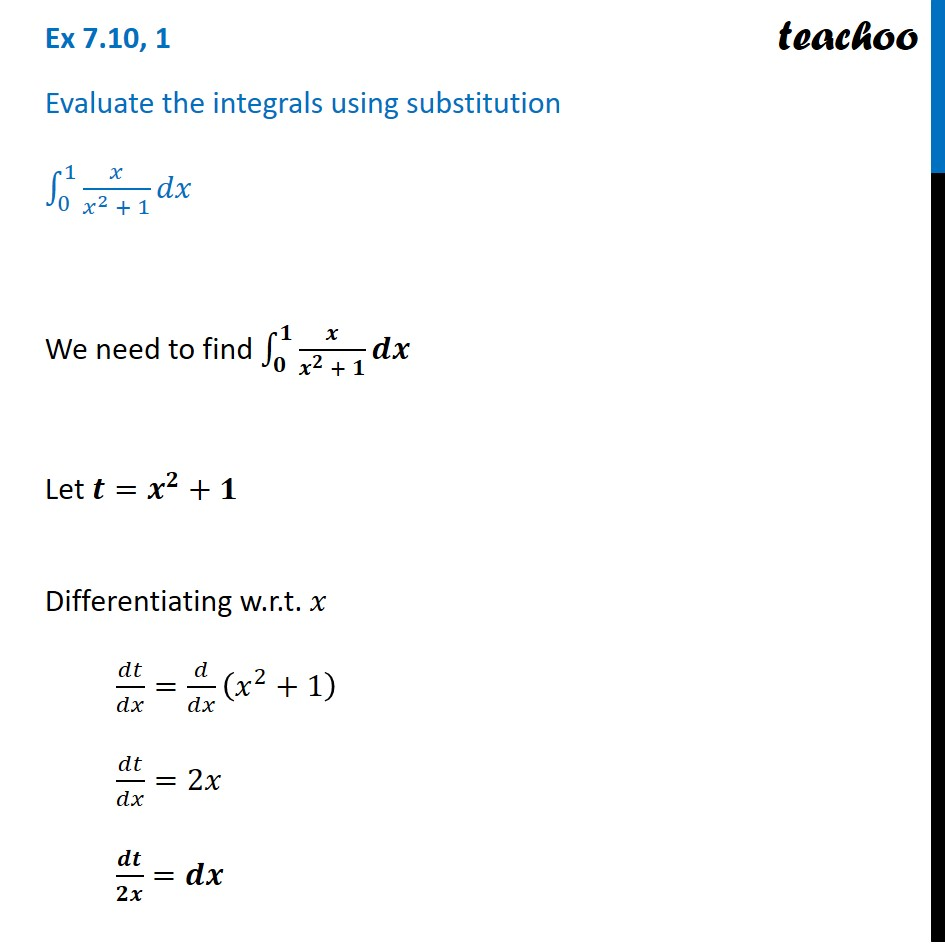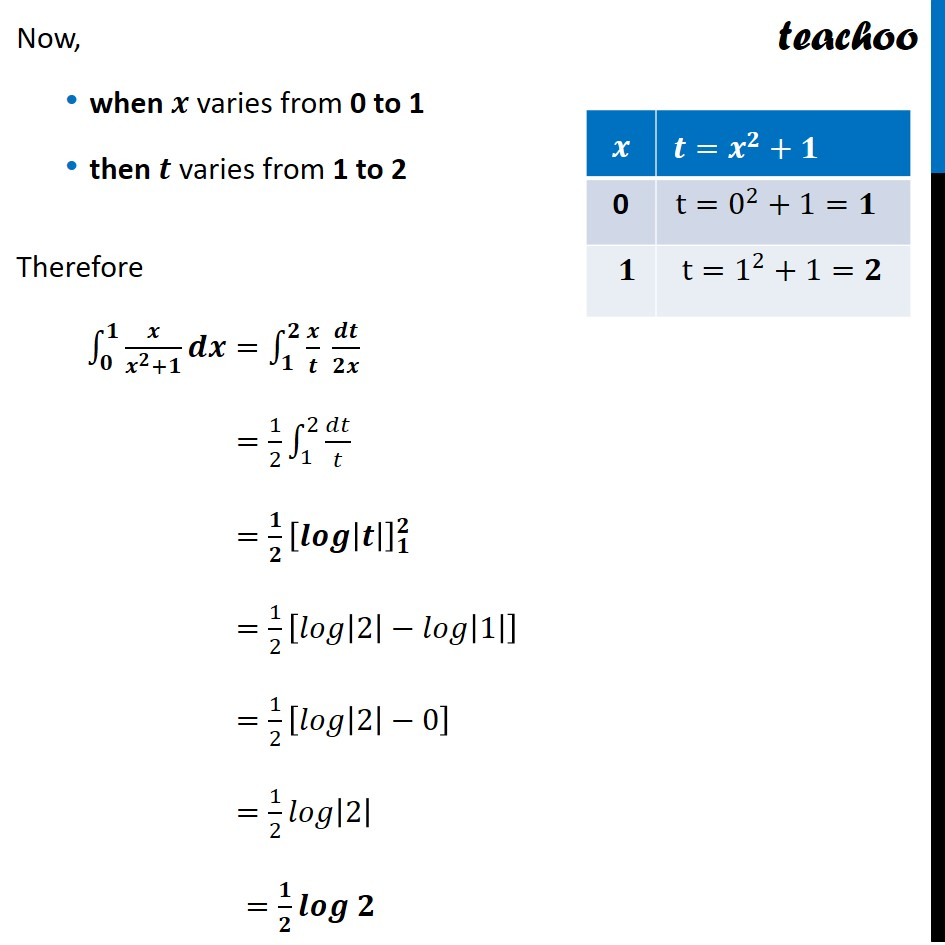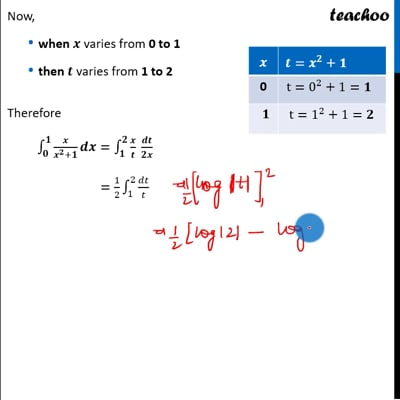Definite Integration - By Substitution

Chapter 7 Class 12 Integrals
Concept wiseThis video is only available for Teachoo black users

Introducing your new favourite teacher - Teachoo Black, at only ₹83 per month

### Transcript

Ex 7.10, 1 Evaluate the integrals using substitution ∫_0^1▒〖𝑥/(𝑥^2 + 1) 𝑑𝑥〗 We need to find ∫_𝟎^𝟏▒〖𝒙/(𝒙^𝟐 + 𝟏) 𝒅𝒙〗 Let 𝒕=𝒙^𝟐+𝟏 Differentiating w.r.t. 𝑥 𝑑𝑡/𝑑𝑥=𝑑/𝑑𝑥 (𝑥^2+1) 𝑑𝑡/𝑑𝑥=2𝑥 𝒅𝒕/𝟐𝒙=𝒅𝒙 Now, when 𝒙 varies from 0 to 1 then 𝒕 varies from 1 to 2 Therefore ∫_𝟎^𝟏▒〖𝒙/(𝒙^𝟐+𝟏) 𝒅𝒙=∫_𝟏^𝟐▒〖𝒙/𝒕 𝒅𝒕/𝟐𝒙〗〗 =1/2 ∫_1^2▒𝑑𝑡/𝑡 =𝟏/𝟐 [𝒍𝒐𝒈|𝒕|]_𝟏^𝟐 =1/2 [𝑙𝑜𝑔|2|−𝑙𝑜𝑔|1|] =1/2 [𝑙𝑜𝑔|2|−0] =1/2 𝑙𝑜𝑔|2| =𝟏/𝟐 𝒍𝒐𝒈 𝟐